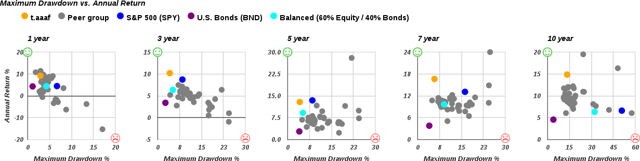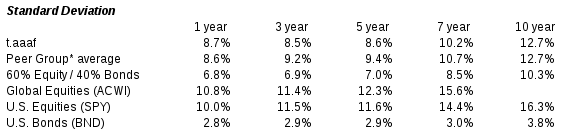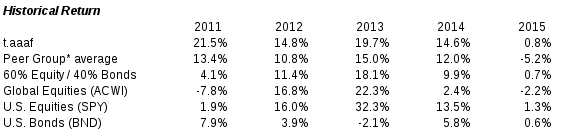## Summary

The Adaptive Asset Allocation (AAA) portfolio combines two different tactical approaches (momentum and minimum variance) into one algorithm.
The intention of this portfolio recipe is to optimize risk-adjusted returns by combining two approaches.
Michael Kapler implemented a version of this portfolio algorithm in 2012 and published his results.
We have updated the analysis and backtesting using current data.
The AAA portfolio has returned 14.8% per year over the past 10 years with a maximum drawdown of 13.4% over that same period.
Adaptive Asset Allocation (AAA) holds an alluring prospect for tactical investors: a nimble portfolio with a risk-adjusted return than beats the benchmarks. By combining two different tactical approaches (momentum and minimum variance) into one algorithm, the adaptive approach builds a portfolio that responds to market conditions with the promise of lower risk.
But does Adaptive Allocation really work? To answer this, let's first understand the analytical basis for this approach, and then we can dive into the results and risk-adjusted returns.
The analytical basis for the Adaptive Asset Allocation Portfolio
In 2012, Macquarie Private Wealth published a paper entitled "Adaptive asset allocation: A primer" using data through May 8, 2012. This paper described a few possible implementations of the methodology. David Varadi then discussed the robustness of this algorithm on his blog, CSSA: New Concepts in Quantitative Research.
In August 2012, Michael Kapler posted his own implementation of Adaptive Asset Allocation on the Systematic Investor Blog. Kapler used the R programming language to implement a version of the AAA algorithm that used 10 asset class ETFs and was rebalanced monthly.
What's the methodology?
The adaptive asset allocation algorithm (or "portfolio recipe") uses two distinct mechanisms to choose assets and percentage allocations for the portfolio.
1. Momentum. This is defined by the total return over the past 180 trading days.
2. Minimum variance. According to the Macquarie paper, "The minimum variance algorithm takes into account the volatility and correlations between the Top 5 assets to create the momentum portfolio with the lowest expected portfolio level volatility." This mechanism uses volatility to choose the asset allocation each month, as defined by the standard deviation over the past 20 trading days.

What's the monthly update process?
Let's look in more detail at the step-by-step process for how the algorithm selects the investable assets each month.
1. Choose the asset universe. This is the set of assets that we will choose from to create the portfolio. Kapler's version of the Adaptive Asset Allocation Portfolio used 10 exchange-traded funds representing global asset classes (SPY, EFA, EWJ, EEM, IYR, RWX, IEF, TLT, DBC, GLD). We have simplified this and our analysis uses a set of nine global asset classes (SPY, EFA, EEM, QQQ, DBC, GLD, TLT, IWM, IYR).
2. Calculate total return (including dividends) for each ETF in the asset universe over the past 180 trading days. Then rank the ETFs from highest to lowest return.
3. Choose the top five ETFs based on their total return over the past 180 trading days. The five chosen ETFs will be the ingredients for the portfolio, but the percentage allocation to each ETF is not yet defined.
4. For each of the five chosen ETFs in this portfolio recipe, apply a volatility metric to determine how much of each ETF to buy. Each ETF will receive a percentage weighting, and the sum of the weightings will be 100%. The percentage weightings are calculated such that the portfolio's volatility is minimized. This is done using a minimum variance algorithm that uses standard deviation with a look-back period of 20 trading days.
5. At the end of each month, re-run the algorithm to create a new list of the top five ETFs and the percentage allocation to each. Then rebalance the portfolio holdings to match the percentages from the updated portfolio recipe.

This portfolio recipe uses monthly rebalancing, which makes it viable as a tactical, do-it-yourself recipe which can be used by individual investors or financial advisors.
The Results: how has the portfolio performed?
We have been tracking variations of the Adaptive Asset Allocation Portfolio since 2014 at recipeinvesting.com. Our backtests extend back to 2003. The particular variation discussed here is shown on recipeinvesting.com as portfolio "t.aaaf"
Over the past 12 months (ending October 31, 2016) the portfolio's total return is 9.2%. Over the past five years, the portfolio has a total return of 12.9% versus the S&P 500's total return of 5.9%. A balanced portfolio of 60% equities and 40% bonds has returned 7.0% over the past five years.
Exhibit A (below) shows the normalized return of the Adaptive Allocation Portfolio against benchmarks for the past five years. The Adaptive Allocation Portfolio (t.aaaf, in yellow) keeps pace with the S&P 500. We have used an ETF (NYSEARCA:SPY) as a proxy for the S&P 500.
Exhibit A: 5-year total return vs. key benchmarks
Now let's look at risk-adjusted returns. Exhibit B (below) plots the various tactical portfolio recipes that we track at recipeinvesting.com. Each dot represents one portfolio recipe, plotted according to its risk (horizontal axis) and annualized total return (vertical axis).
The green smiley face marks the fabled and elusive "northwest corner," where a perfect low-risk, high-return portfolio would be plotted. Since that perfect portfolio doesn't exist, instead we look for portfolios that are closest to the top left corner.
The yellow dot shows the total return and risk for the Adaptive Asset Allocation Portfolio recipe. For each time period, the AAA portfolio is one of the better choices since it appears closer to the top left corner.
The dark blue dot shows the S&P 500. Note that the AAA portfolio has generated higher return with lower risk over all periods except the 5-year period when the AAA portfolio return was slightly less than SPY.
The purple dot shows U.S. Bonds (NYSEARCA:BND). The light blue dot shows a balanced portfolio recipe consisting of 60% equity and 40% bonds. The risk on these graphs is measured by maximum drawdown (the largest peak to trough loss) over the specified period.
Exhibit B: Risk (horizontal axis) vs. return (vertical axis)We can also look at standard deviation as a measure of volatility. Using this metric, we can see in Exhibit C (below) that the AAA portfolio (in the row labeled t.aaaf) has consistently shown a lower standard deviation than SPY over all time periods. The Peer Group average is the group of tactical, do-it-yourself portfolios tracked by VizMetrics at www.recipeinvesting.com
Exhibit C: Volatility, as measured by Standard Deviation
data through October 31, 2016Exhibit D (below) shows the historical return percentages of the Adaptive Asset Allocation recipe (t.aaaf) along with its peers from 2011 to 2015. t.aaaf has lagged SPY in three of the past five years, but t.aaaf's outperformance in 2011 was notable, when it beat SPY by 19.6% that year.
Exhibit D: Historical annual returnsConclusion
Kapler's Adaptive Asset Allocation methodology showed solid results when he first published in 2012, and we have been able to produce similar results using recent data and a modified set of ETFs -- we use a nine ETFs and Kapler used ten. Our backtested version of the AAA portfolio (t.aaaf) has generated a return of 14.8% over the past 10 years, with less risk than the S&P 500 (using SPY as a proxy). We will continue to track the performance of Adaptive Asset Allocation Portfolio (t.aaaf) at www.recipeinvesting.com.